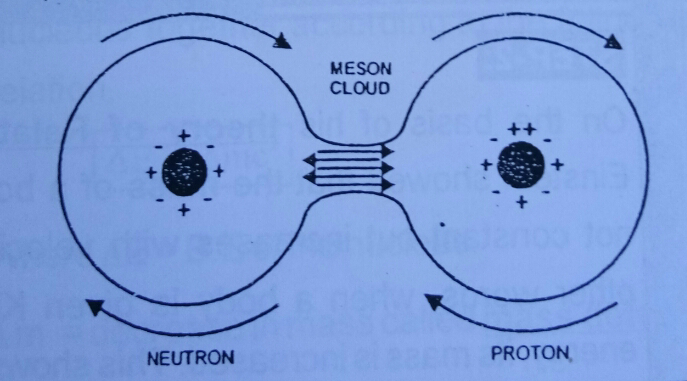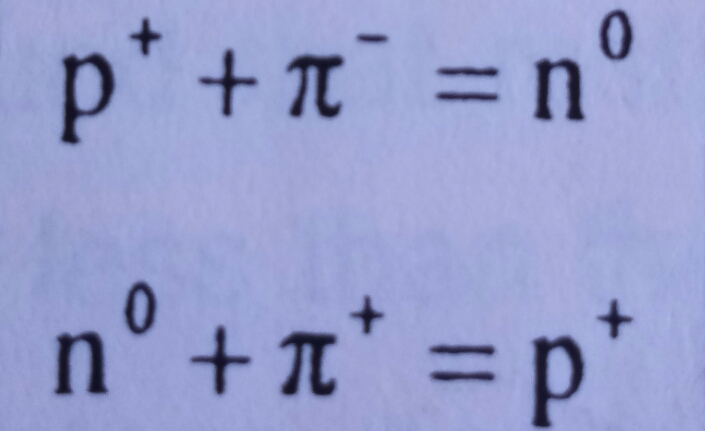# Nuclear Forces - Properties of nuclear forces

Views

## Nuclear forces

Nuclear Forces are the strongest forces of attraction which hold the nucleons means protons and neutrons together in the nucleus of an atom.

Nuclear Forces are very complex in nature. The concept about the nuclear forces was first given by Yukawa, a Japanese physicist   . According to yukawa , Nuclear Forces arise due to exchange of pie- mesons between the nucleons.

👉 visit:Rutherford's Nuclear model

### Nuclear forces

Nature of Nuclear Forces

A Japanese physicist named Yukawa in the year  1936  postulated that the nuclear Forces between Nucleons (Protons and neutrons)  arise due to the continuous exchange of particles called Mesons between the nucleons. Later on, these particles were detected experimentally.

There were three types of mesons which were detected by the physicists in the experiments. These three types of mesons are discussed below :

Neutral  pi-mesons :
These are the types of mesons carrying no charge.

Positive pi-mesons :
These type of mesons carrying  a unit positive charge.

Negative pi-mesons :
These are the types of mesons which carry a unit negative charge.

Also visit 👉: Linearity (waves and optics)

### Nuclear forces

According to Meson Theory or Yukawa theory :

1) According to Meson theory, all the nucleons present inside the nucleus of an atom are made up of an identical cores which are surrounded by a pulsating Cloud of pie-mesons. Check it below in the given figure;Nuclear Force

2) According to this theory, mesons also called as pions can be either neutral or carrying either positive or negative charge.

3)  Difference between proton and neutron depends upon the composition of respective meson Cloud.

4)  According to this theory, the force between proton and neutron is due to the exchange of charged mesons between them. I.e.,Nuclear Forces

Thus nucleons means protons and neutrons continuously exchange their nature by emitting and absorbing pi-mesons.

Nuclear forces

Properties of Nuclear Forces :

1) Nuclear Forces are charge independent:

This means that nuclear Forces can occur between any kind of charges. They can act between a pair of protons, between a pair of neutrons, between a pair of proton and neutron.

2) Nuclear Forces are Short Range Forces:

When the distance between the nucleons is comparable with the size of the nucleus only then nuclear forces can exist. This indicates that the Nuclear Forces are short range forces.

3) Nuclear Forces are non-central :

The force between the nucleons is actually  non central because it doesn't depend upon the line joining their centres. In fact, they are said to be partial non-central nature.

4) Nuclear Forces are spin dependent :

It has been observed that the force between the nucleons having parallel spin is greater than the force between nucleons having anti parallel spin. Thus nuclear Forces are spin dependent.

5) Nuclear Forces are Saturated Forces :

This means that a Nucleon can only form bounds with a certain definite number of its neighbours and no more. In other words, each nucleon interacts with its immediate neighbors only.

6) Magnitude of Nuclear Forces :

The magnitude of Nuclear Forces  is 100 times the magnitude of electrostatic forces .

7) Exchange character:

They posses exchange character because the force existing between the two nucleons is actually the result of exchange of charged mesons between them.

In This article I have covered topics like nuclear forces , nature of nuclear forces and the properties of nuclear forces.